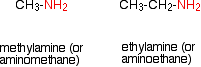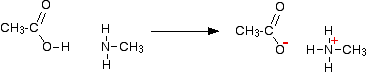# Simple Reactions of Carboxylic Acids as Acids

•• Contributed by Jim Clark
• Former Head of Chemistry and Head of Science at Truro School in Cornwall

This page looks at the simple reactions of carboxylic acids as acids, including their reactions with metals, metal hydroxides, carbonates and hydrogencarbonates, ammonia and amines.

## The acidity of the carboxylic acids

Using the definition of an acid as a "substance which donates protons (hydrogen ions) to other things", the carboxylic acids are acidic because of the hydrogen in the -COOH group. In solution in water, a hydrogen ion is transferred from the -COOH group to a water molecule. For example, with ethanoic acid, you get an ethanoate ion formed together with a hydroxonium ion, H3O+.

This reaction is reversible and, in the case of ethanoic acid, no more than about 1% of the acid has reacted to form ions at any one time. (This is a rough-and-ready figure and varies with the concentration of the solution.)

These are therefore weak acids.

$CH_3COOH + H_2O \rightleftharpoons CH_3COO^- + H_3O^+$

This equation is often simplified by removing the water to:

$CH_3COOH (aq) \rightleftharpoons CH_3COO^- (aq) + H^+$

However, if you are going to use this second equation, you must include state symbols. They imply that the hydrogen ion is actually attached to a water molecule.

## The pH of carboxylic acid solutions

The pH depends on both the concentration of the acid and how easily it loses hydrogen ions from the -COOH group. Ethanoic acid is typical of the acids where the -COOH group is attached to a simple alkyl group. Typical lab solutions have pH's in the 2 - 3 range, depending on their concentrations.

Methanoic acid is rather stronger than the other simple acids, and solutions have pH's about 0.5 pH units less than ethanoic acid of the same concentration.

## Reactions of carboxylic acids with metals

Carboxylic acids react with the more reactive metals to produce a salt and hydrogen. The reactions are just the same as with acids like hydrochloric acid, except they tend to be rather slower.

For example, dilute ethanoic acid reacts with magnesium. The magnesium reacts to produce a colorless solution of magnesium ethanoate, and hydrogen is given off. If you use magnesium ribbon, the reaction is less vigorous than the same reaction with hydrochloric acid, but with magnesium powder, both are so fast that you probably wouldn't notice much difference.

$2CH_3COOH + Mg \rightarrow (CH_3COO)_2Mg + H_2$

## Reactions of carboxylic acids With metal hydroxides

These are simple neutralisation reactions and are just the same as any other reaction in which hydrogen ions from an acid react with hydroxide ions. They are most quickly and easily represented by the equation:

$H^+ (aq) + OH^- \rightarrow H_2O(l)$

If you mix dilute ethanoic acid with sodium hydroxide solution, for example, you simply get a colorless solution containing sodium ethanoate. The only sign that a change has happened is that the temperature of the mixture will have increased.

This change could well be represented by the ionic equation above, but if you want it, the full equation for this particular reaction is:

$CH_3COOH + NaOH \rightarrow CH_3COONa + H_2O$

## Reactions of carboxylic acids with carbonates and hydrogencarbonates

In both of these cases, a salt is formed together with carbon dioxide and water. Both are most easily represented by ionic equations.

For carbonates:

$2H^+ (aq) + CO_3^{2-} \rightarrow H_2O (l) + CO_2 (g)$

. . . and for hydrogencarbonates:

$H^+ (aq) + HCO_3^{-} \rightarrow H_2O (l) + CO_2 (g)$

If you pour some dilute ethanoic acid onto some white sodium carbonate or sodium hydrogencarbonate crystals, there is an immediate fizzing as carbon dioxide is produced. You end up with a colorless solution of sodium ethanoate.

With sodium carbonate, the full equation is:

$2CH_3COOH + Na_2CO_3 \rightarrow 2CH_3COONa + H_2O + CO_2$

. . . and for sodium hydrogencarbonate:

$CH_3COOH + NaHCO_3 \rightarrow CH_3COONa + H_2O + CO_2$

There is very little obvious difference in the vigor of these reactions compared with the same reactions with dilute hydrochloric acid. However, you would notice the difference if you used a slower reaction - for example with calcium carbonate in the form of a marble chip. With ethanoic acid, you would eventually produce a colorless solution of calcium ethanoate.

$2CH_3COOH + CaCO_3 \rightarrow (CH_3COO)_2Ca + H_2O + CO_2$

In this case, the marble chip would react noticeably more slowly with ethanoic acid than with hydrochloric acid.

## Reactions of carboxylic acids with ammonia

Ethanoic acid reacts with ammonia in exactly the same way as any other acid does. It transfers a hydrogen ion to the lone pair on the nitrogen of the ammonia and forms an ammonium ion.If you mix together a solution of ethanoic acid and a solution of ammonia, you will get a colorless solution of ammonium ethanoate.

$CH_3COOH + NH_3 \rightarrow CH_3COONH_4$

## Reactions of carboxylic acids with amines

Amines are compounds in which one or more of the hydrogen atoms in an ammonia molecule have been replaced by a hydrocarbon group such as an alkyl group. For simplicity, we'll just look at compounds where only one of the hydrogen atoms has been replaced. These are called primary amines.

Simple primary amines include:The small amines are very similar indeed to ammonia in many ways. For example, they smell very much like ammonia and are just as soluble in water. Because all you have done to an ammonia molecule is swap a hydrogen for an alkyl group, the lone pair is still there on the nitrogen atom.

That means that they will react with acids (including carboxylic acids) in just the same way as ammonia does. For example, ethanoic acid reacts with methylamine to produce a colorless solution of the salt methylammonium ethanoate.However complicated the amine, because all of them have got a lone pair on the nitrogen atom, you would get the same sort of reaction.

## Contributors

Jim Clark (Chemguide.co.uk)